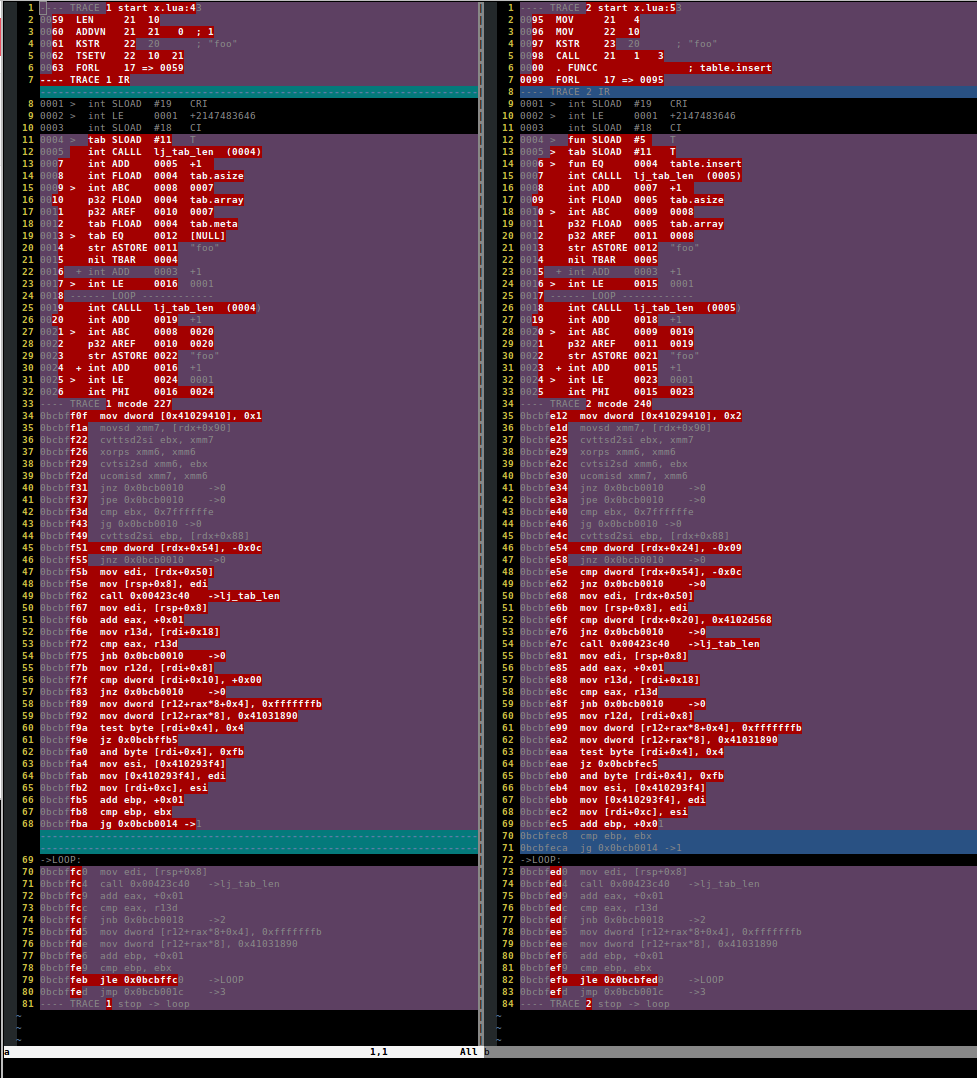Lua table 如何实现最快的 insert?

Fastest Table Inserts

Posted by ms2008 on March 10, 2020

function _M.table_keys(self, tb)
if type(tb) ~= "table" then
ngx.log(ngx.WARN, type(tb) .. "was given to table_keys")
return tb
end

local t = {}
for key,_ in pairs(tb) do
table.insert(t, key)
end

return t
end

CASE 1

local t = {}
local table_insert = table.insert

for i=1,1e7 do
table_insert(t, i)
end

CASE 2

Short inline expressions can be faster than function calls. t[#t+1] = 0 is faster than table.insert(t, 0).

local t = {}

for i=1,1e7 do
t[#t+1] = i
endlocal t = {}
local c = 1

for i=1,1e7 do
t[c] = i
c = c + 1
end

CASE 4

CASE-3 的性能已经非常好了，但还是漏了一个优化的点：table 的扩容。

table 的扩容用的是 hashpow2，它是不小于 table hash or array 区域数量的 2^n^ 形式的整数

local table_new = require "table.new"

local t = table_new(1e7, 0)
local c = 1

for i=1,1e7 do
t[c] = i
c = c + 1
end

参考文献